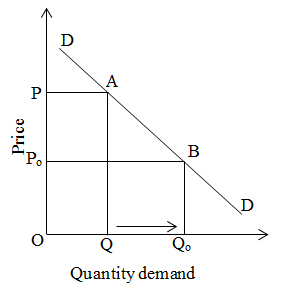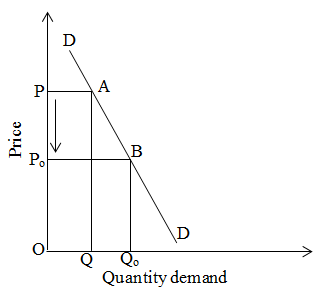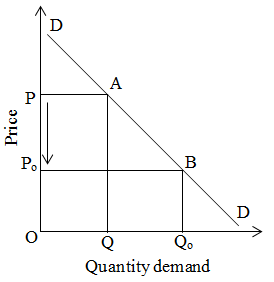# Measurement of price elasticity of demand by total outlay method

This method is first propounded by prof. Alferd Marshall. In this method, we will compare total expenditure made by consumer after and before change in price of commodity. Here, we can’t actually measure the certain percentage change due to percentage change in price. We can measure only elasticity is greater than one, less than one or equal to one. Under this method we will explain different three types as follows:

1. Elasticity of demand greater than one:

If the expenditure made by the consumer is increases due to the fall of price of commodity, then it is known as elasticity of demand greater than one. We can explain it by given figure:

 Price (P) Quantity (Q) T.E = P*Q 5 20 5*20=100 4 30 4*30=120

We can explain it by the given figure:On the above figure, when price is OP, quantity demand is OQ. At that time total expenditure (T.E) is OPAQ. When the price decreases from P to Po then quantity demand is OQo, at this time T.E is OPoBQo .
At this time T.E of OPoBQo > T.E of OPAQ.

2. Elasticity of demand less than one

If the total expenditure made by consumer decreases due to fall in price is known as elasticity of demand less than one.

 Price (P) Quantity (Q) T.E = P*Q 5 20 5*20 = 100 4 22 4*22 = 88

We can explain it by the given figure:On the above figure, price is OP and quantity demand is OQ. When price decreases from P to po then quantity demand is Qo. In initial stage T.E is OPAB and later it is OPoBQo. In this situation, T.E of OPoBQo < T.E of OPAB.

3. Elasticity of demand equal to one

If the total expenditure made by consumers remains constant due to any change in price of any commodity is called elasticity of demand equal to one. We can explain by the given table:

 Q Quantity (Q) TE 5 20 100 4 25 100

Same concept can be explained by figure:On the above figure, in initial stage price is OP and quantity demand is OQ. In this condition total expenditure is OPAQ. When price decreases from p to Po then quantity demand increases from Q to Qo. In this condition TE is OPoBQo. In this situation TE of OPAQ = TE of OPoBQo.